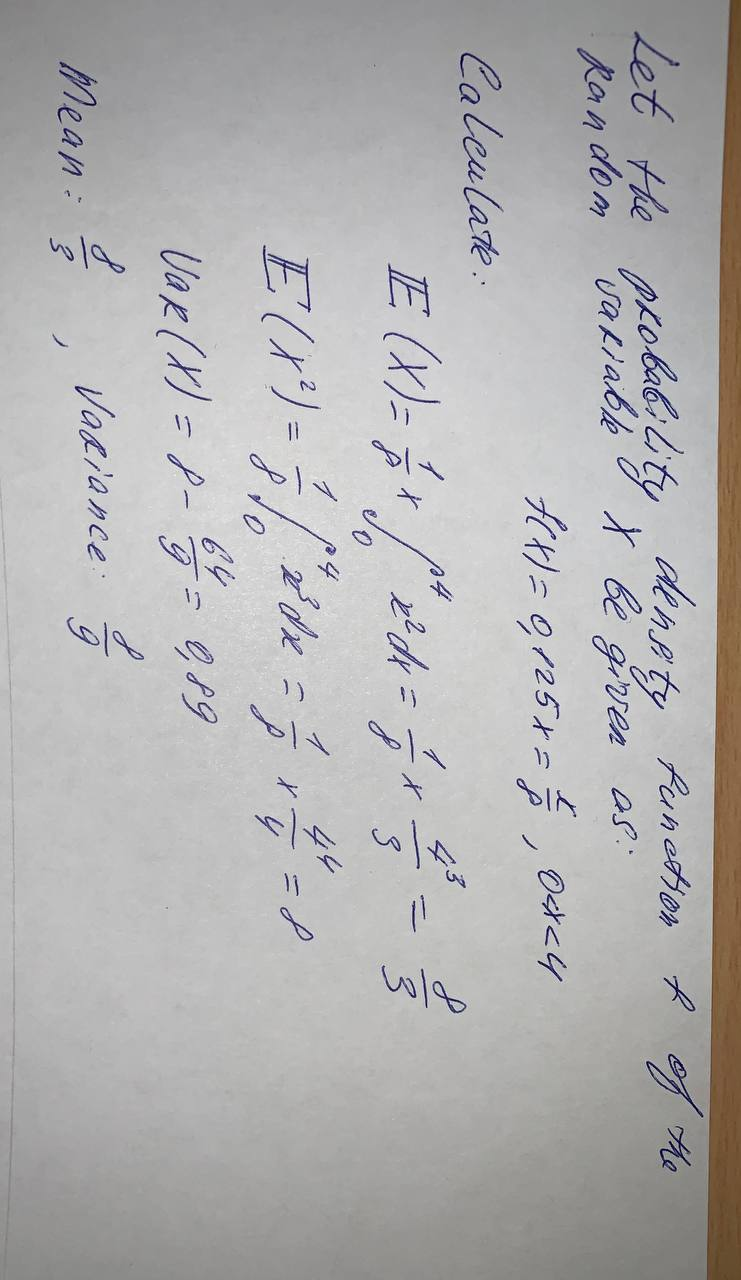# Suppose that f(x)=0,125x \text{ for } 0<x<4. Determine the mean and variance of X.York 2021-09-23 Answered

Suppose that $$\displaystyle{f{{\left({x}\right)}}}={0},{125}{x}\ \text{ for }\ {0}{<}{x}{<}{4}$$. Determine the mean and variance of X.

• Questions are typically answered in as fast as 30 minutes

### Plainmath recommends

• Get a detailed answer even on the hardest topics.
• Ask an expert for a step-by-step guidance to learn to do it yourself.sovienesY

At the given condition: